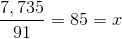## Example Questions

### Example Question #1 : How To Divide Odd Numbers

Choose the answer which best solves the equation below: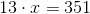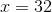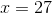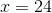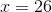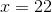Explanation:

There are two ways to solve this problem. First you can do so algebraically by dividing both sides by 13: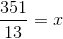But, there is another way, which if you understand odd numbers, is even faster. Of all the answers above, only one is odd. You know, given the equation, thatmust be odd--any odd number multiplied by an odd number will yeild an odd number.  If you multiply an odd number by an even number, you will get an even number.

### Example Question #2 : How To Divide Odd Numbers

Solve forin the following equation: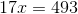Explanation:

There are two ways to approach this problem:

1. Use the rule that states that any two odd numbers multiplied together will yield another odd number.

Using this rule, only one answer is an odd number (29) which will yield another odd number (493) when multiplied by the given odd number (17).

2. Solve algebraically: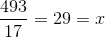### Example Question #3 : How To Divide Odd Numbers

Solve forin the follwing equation: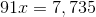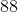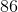Explanation:

There are two ways to approach this problem:

1. Use the rule that states that any two odd numbers multiplied together will yield another odd number.

Using this rule, only one answer is an odd number (85) which will yield another odd number (7,735) when multiplied by the given odd number (91).

2. Solve algebraically: